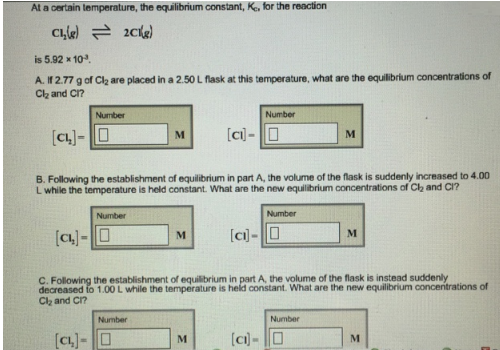# Problem: At a certain temperature, the equilibrium constant, K c, for the reaction Cl2(g) ⇌ 2Cl(g) is 5.92 x 104. A. If 2.77 g of Cl 2 are placed in a 2.50 L flask at this temperature, what are the equilibrium concentrations of Cl2 and Cl? B. Following the establishment of equilibrium in part A, the volume of the flask is suddenly increased to 4.00 L while the temperature is held constant. What are the new equilibrium concentrations of Cl2 and Cl? C. Following the establishment of equilibrium in part A, the volume of the flask is instead suddenly decreased to 1.00 L while the temperature is held constant. What are the new equilibrium concentrations of Cl2 and Cl?

🤓 Based on our data, we think this question is relevant for Professor Du's class at UGA.

###### FREE Expert Solution###### Problem Details

At a certain temperature, the equilibrium constant, K c, for the reaction

Cl2(g) ⇌ 2Cl(g)

is 5.92 x 104

A. If 2.77 g of Cl 2 are placed in a 2.50 L flask at this temperature, what are the equilibrium concentrations of Cl2 and Cl?

B. Following the establishment of equilibrium in part A, the volume of the flask is suddenly increased to 4.00 L while the temperature is held constant. What are the new equilibrium concentrations of Cl2 and Cl?

C. Following the establishment of equilibrium in part A, the volume of the flask is instead suddenly decreased to 1.00 L while the temperature is held constant. What are the new equilibrium concentrations of Cl2 and Cl?